# HashMap底层实现原理

### HashMap概述

HashMap实现了Map接口，我们常用HashMap进行put和get操作读存键值对数据。下面介绍基于jdk1.8深入了解HashMap底层原理。

### HashMap数据结构

HashMap实际是一种“数组+链表”数据结构。在put操作中，通过内部定义算法寻止找到数组下标，将数据直接放入此数组元素中，若通过算法得到的该数组元素已经有了元素（俗称hash冲突，链表结构出现的实际意义也就是为了解决hash冲突的问题）。将会把这个数组元素上的链表进行遍历，将新的数据放到链表末尾。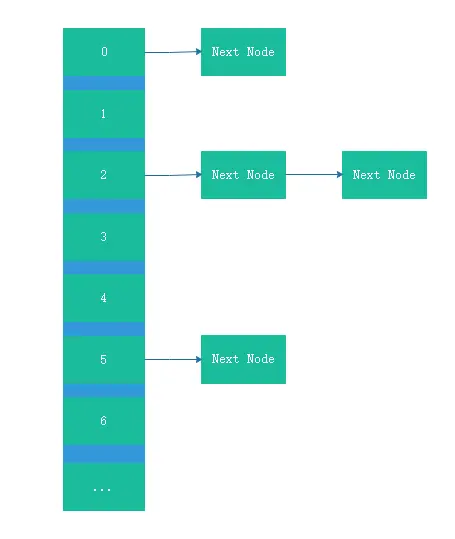#### 存储数据的对象

``````static class Node<K,V> implements Map.Entry<K,V> {
final int hash;
final K key;
V value;
Node<K,V> next;
}``````

hash：通过hash算法的出来的值。hash值的算法我们看下HashMap源代码的实现

``````static final int hash(Object key) {
int h;
return (key == null) ? 0 : (h = key.hashCode()) ^ (h >>> 16);
}``````

String.hashCode()

``````public int hashCode() {
int h = hash;
if (h == 0 && value.length > 0) {
char val[] = value;

for (int i = 0; i < value.length; i++) {
h = 31 * h + val[i];
}
hash = h;
}
return h;
}``````

Integer.hashCode()

``````public static int hashCode(int value) {
return value;
}``````

key：存储数据的key

value：存储数据的value

next：下一个数据，出现哈希冲突时，该数组元素会出现链表结构，会使用next指向链表中下一个元素对象

### HashMap put存储数据是如何处理的

#### HashMap有几个重要的变量

``````transient Node<K,V>[] table;
int threshold;
int modCount;
int size;``````

table：存储数组的变量，初始长度为16通过源代码看出在第一次进行resize扩容（java是静态语言，在定义数组初始化时，需要定义数组的长度，在map数据增长后，内部机制会进行重新定义一个数组做到扩容的操作）初始化时，会将默认静态变量

``static final int DEFAULT_INITIAL_CAPACITY = 1 << 4;``

modCount：记录内部结构发生变化的次数，put操作（覆盖值不计算）以及其他...

size：实际存储的元素数量

#### put的流程

``````final V putVal(int hash, K key, V value, boolean onlyIfAbsent,
boolean evict) {
Node<K,V>[] tab; Node<K,V> p; int n, i;
// 判断数组是否为空，长度是否为0，是则进行扩容数组初始化
if ((tab = table) == null || (n = tab.length) == 0)
n = (tab = resize()).length;
// 通过hash算法找到数组下标得到数组元素，为空则新建
if ((p = tab[i = (n - 1) & hash]) == null)
tab[i] = newNode(hash, key, value, null);
else {
Node<K,V> e; K k;
// 找到数组元素，hash相等同时key相等，则直接覆盖
if (p.hash == hash &&
((k = p.key) == key || (key != null && key.equals(k))))
e = p;
// 该数组元素在链表长度>8后形成红黑树结构的对象
else if (p instanceof TreeNode)
e = ((TreeNode<K,V>)p).putTreeVal(this, tab, hash, key, value);
else {
// 该数组元素hash相等，key不等，同时链表长度<8.进行遍历寻找元素，有就覆盖无则新建
for (int binCount = 0; ; ++binCount) {
if ((e = p.next) == null) {
// 新建链表中数据元素
p.next = newNode(hash, key, value, null);
if (binCount >= TREEIFY_THRESHOLD - 1) // -1 for 1st
// 链表长度>=8 结构转为 红黑树
treeifyBin(tab, hash);
break;
}
if (e.hash == hash &&
((k = e.key) == key || (key != null && key.equals(k))))
break;
p = e;
}
}
if (e != null) { // existing mapping for key
V oldValue = e.value;
if (!onlyIfAbsent || oldValue == null)
e.value = value;
afterNodeAccess(e);
return oldValue;
}
}
++modCount;
if (++size > threshold)
resize();
afterNodeInsertion(evict);
return null;
}``````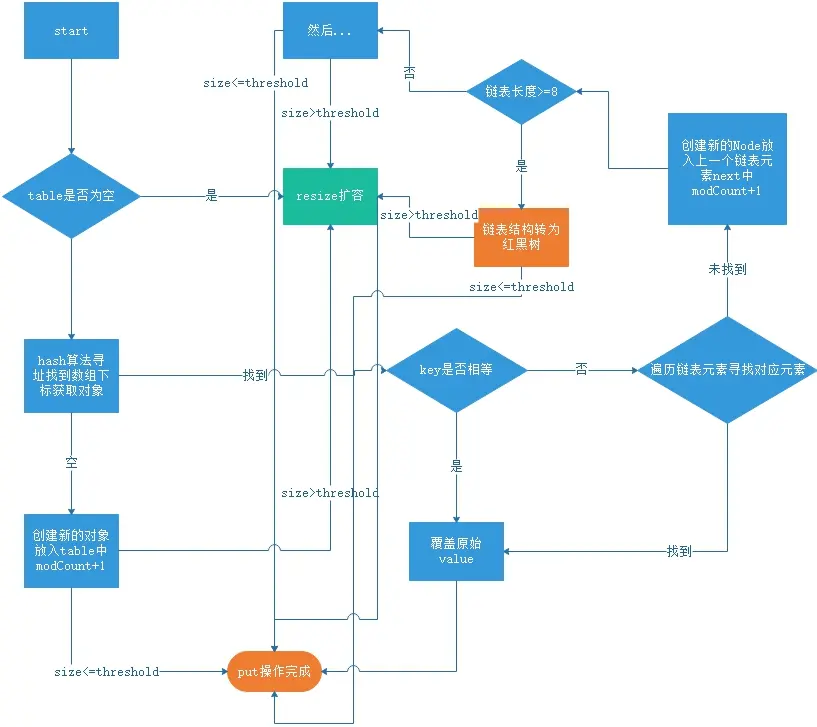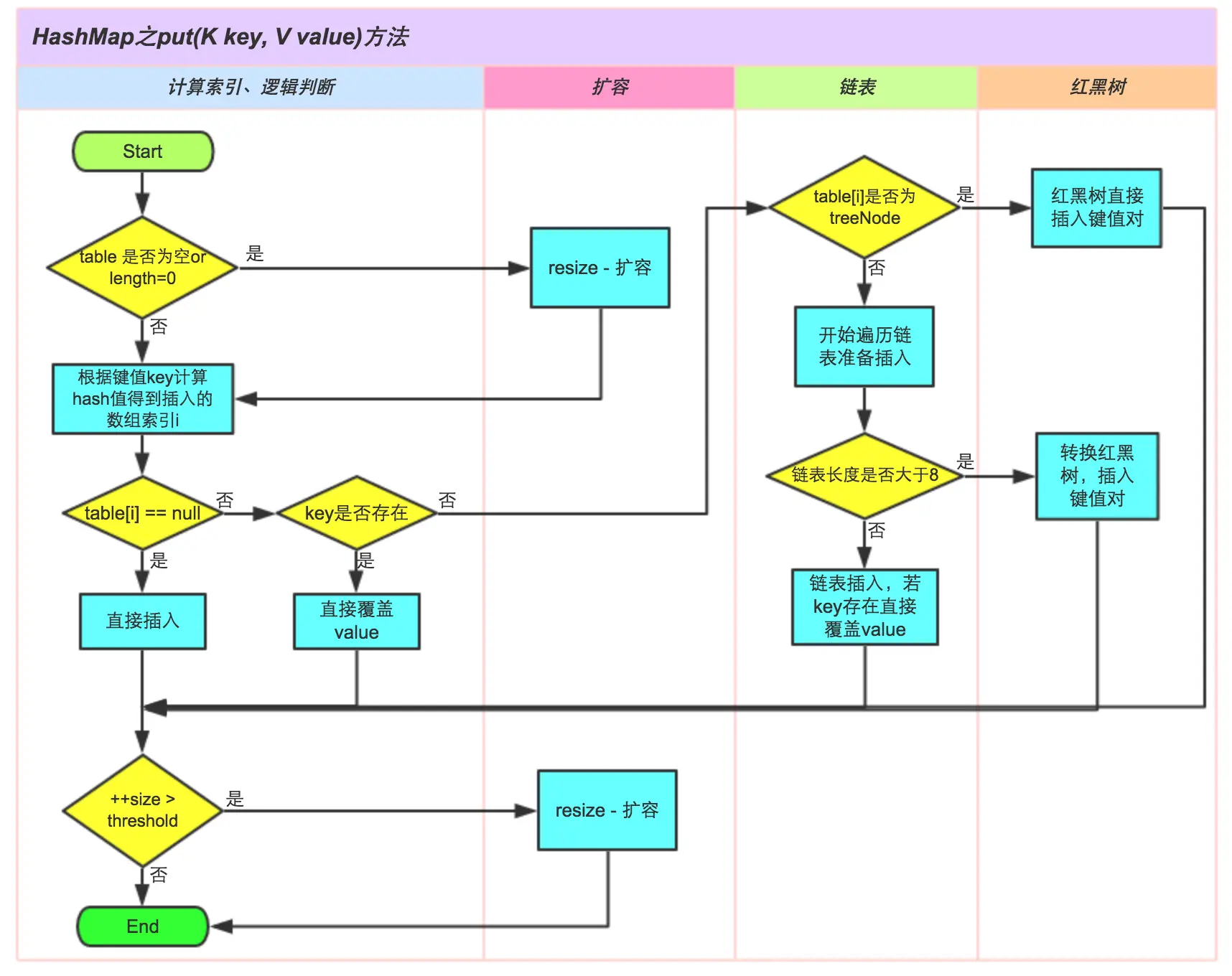1、首选判断table是否为空，数组长度为空，将会进行第一次初始化。（在实例化HashMap是，并不会进行初始化数组）

2、进行第一次resize()扩容之后。开始通过hash算法寻址找到数组下标。若数组元素为空，则创建新的数组元素。若数组元素不为空，同时hash相等，key不相等，同时不是TreeNode数据对象，将遍历该数组元素下的链表元素。若找到对应的元素，则覆盖，如果没有找到，就新建元素，放入上一个链表元素的next中，在放入元素之后，如果条件满足"链表元素长度>8"，则将该链表结构转为"红黑树结构"。

3、找到对应的数组元素或者链表元素，同时创建新的数据元素或者覆盖元素之后。如果条件满足元素大小size>允许的最大元素数量threshold，则再一次进行扩容操作。每次扩容操作，新的数组大小将是原始的数组长度的两倍。

4、put操作完成。

#### 调用put方法示例

``````HashMap<Integer, String> hashMap = new HashMap<Integer, String>(4, 0.75f);// 1
int a1 = 1;
int a2 = 2;
int a3 = 5;
System.out.println(String.valueOf(a1&3) + " " + String.valueOf(a2&3)+ " " + String.valueOf(a3&3));// 1 2 1 数组下标
hashMap.put(a1, "1");// 2
hashMap.put(a2, "2");// 3
hashMap.put(a3, "5");// 4``````

1、创建了一个HashMap对象，初始化initialCapacity为4，增长因子为0.75。threshold初始化为4

2、进行了第一次put，因为table为空，进行了第一次resize()扩容操作，数组进行初始化，默认为16. threshold变为3。同时通过hash算法（数组长度n-1 & hash）即为1。

3、第二次put操作，同时获取数组下标为2，此时数组下标为2当前没有数组元素，则直接创建数据元素放入

4、第三次put操作，得到数组下标为1已经有了一个数组元素。同时我们知道存储数据的Node对象中又一个next，则新的此时的数据元素放入上一个链表中next为空的Node中的next中。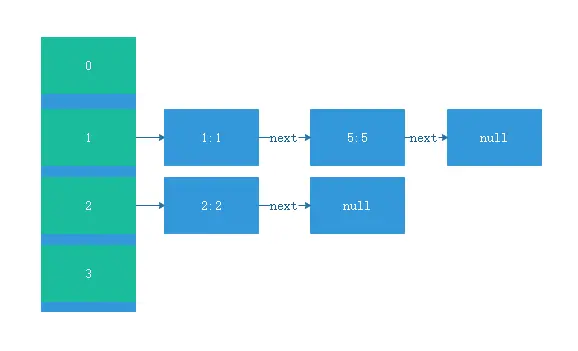#### HashMap扩容resize()

java是静态方法，在数组进行初始化时，必须给一个数组长度。HashMap定义默认的数组长度为16。条件满足元素size>允许的最大元素数量threshold。则进行扩容。一般来说，在put操作中，HashMap至少进行了一次扩容（第一次为初始化）。

``````int a4 = 6;
hashMap.put(a4, "6");``````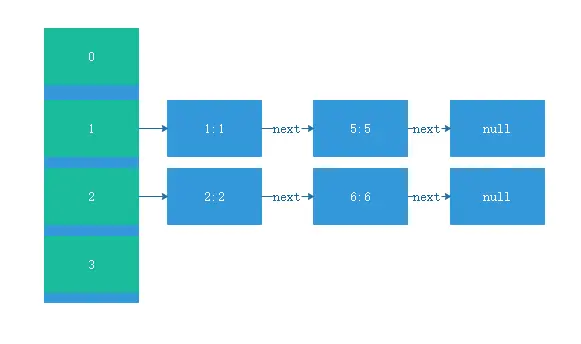``````final Node<K,V>[] resize() {
Node<K,V>[] oldTab = table;
int oldCap = (oldTab == null) ? 0 : oldTab.length;
int oldThr = threshold;
int newCap, newThr = 0;
if (oldCap > 0) {
// 超过最大限制，不进行扩容
if (oldCap >= MAXIMUM_CAPACITY) {
threshold = Integer.MAX_VALUE;
return oldTab;
}
// 进行原始长度*2扩容
else if ((newCap = oldCap << 1) < MAXIMUM_CAPACITY &&
oldCap >= DEFAULT_INITIAL_CAPACITY)
newThr = oldThr << 1; // double threshold
}
// 第一次初始化
else if (oldThr > 0) // initial capacity was placed in threshold
newCap = oldThr;
else {               // zero initial threshold signifies using defaults
newCap = DEFAULT_INITIAL_CAPACITY;
}
// 第一次初始化
if (newThr == 0) {
float ft = (float)newCap * loadFactor;
newThr = (newCap < MAXIMUM_CAPACITY && ft < (float)MAXIMUM_CAPACITY ?
(int)ft : Integer.MAX_VALUE);
}
// 新的最大允许元素数量值
threshold = newThr;
@SuppressWarnings({"rawtypes","unchecked"})
// 新的数组
Node<K,V>[] newTab = (Node<K,V>[])new Node[newCap];
table = newTab;
if (oldTab != null) {
// 遍历老数组
for (int j = 0; j < oldCap; ++j) {
Node<K,V> e;
if ((e = oldTab[j]) != null) {
oldTab[j] = null;
// 直接按照原始索引放入新数组中
if (e.next == null)
newTab[e.hash & (newCap - 1)] = e;
else if (e instanceof TreeNode)
((TreeNode<K,V>)e).split(this, newTab, j, oldCap);
else { // preserve order
// 遍历链表
Node<K,V> loHead = null, loTail = null;
Node<K,V> hiHead = null, hiTail = null;
Node<K,V> next;
do {
next = e.next;
//　放入原索引
if ((e.hash & oldCap) == 0) {
if (loTail == null)
else
loTail.next = e;
loTail = e;
}
// 原索引＋oldCap
else {
if (hiTail == null)
else
hiTail.next = e;
hiTail = e;
}
} while ((e = next) != null);
if (loTail != null) {
loTail.next = null;
}
if (hiTail != null) {
hiTail.next = null;
}
}
}
}
}
return newTab;
}``````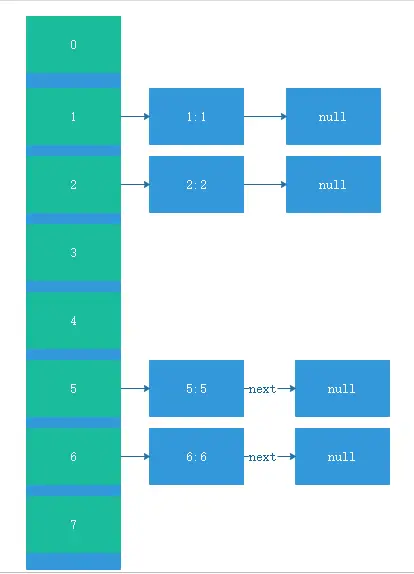``````1(1:1 == > 5:5)

2(2:2 == > 6:6)``````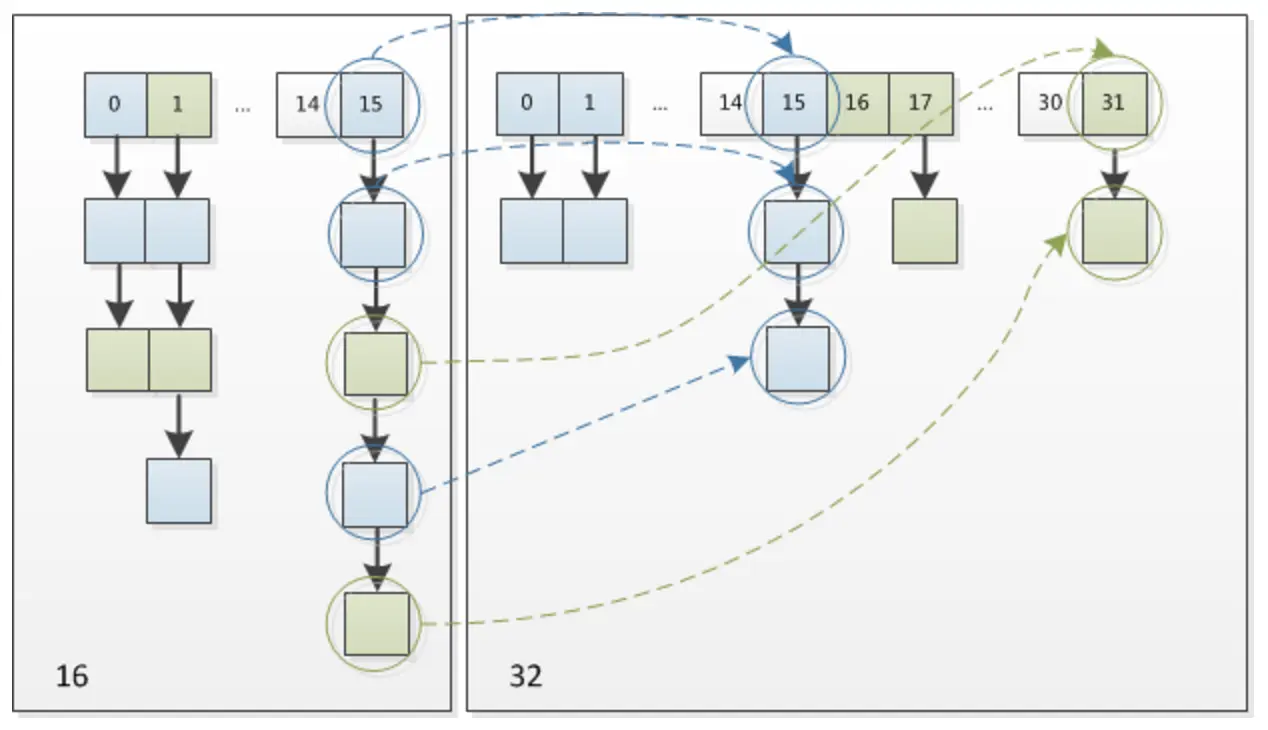### get寻找数据

``````final Node<K,V> getNode(int hash, Object key) {
Node<K,V>[] tab; Node<K,V> first, e; int n; K k;
if ((tab = table) != null && (n = tab.length) > 0 &&
(first = tab[(n - 1) & hash]) != null) {
if (first.hash == hash && // always check first node
((k = first.key) == key || (key != null && key.equals(k))))
return first;
if ((e = first.next) != null) {
if (first instanceof TreeNode)
return ((TreeNode<K,V>)first).getTreeNode(hash, key);
do {
if (e.hash == hash &&
((k = e.key) == key || (key != null && key.equals(k))))
return e;
} while ((e = e.next) != null);
}
}
return null;
}``````

get方法比较简单，基本流程为通过key的hashCode和寻址算法得到数组下标，若数组元素中的key和hash相等，则直接返回。若不想等，同时存在链表元素，则遍历链表元素进行匹配。由于1.8引用了红黑树结构，在链表元素过多时，1.8的实现将比1.7在get和put操作上效率高上很多。

【我是码农，也不是码农】软件 – 注重思想与逻辑